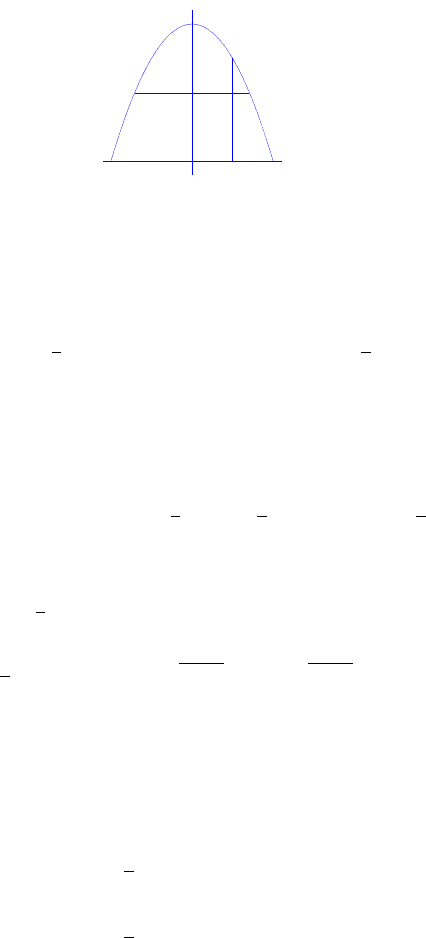# STAT330 Lecture Notes - Lecture 2: Marginal Distribution

53 views4 pages
School
Department
Course
Professor20.(a) The support of (X, Y ) is pictured below:
x
x
y
y
0
1
1−1
(x,1−x2)
(sqrt(1−y),y)(−sqrt(1−y),y)
y=1−x2
1 =
Z
−∞
Z
−∞
f(x, y)dxdy =k
1
Z
x=1
1x2
Z
y=0 x2+ydydx
=k
1
Z
1x2y+1
2y2|1x2
0dx =k
1
Z
1x21x2+1
21x22dx
=k
1
Z
0h2x21x2+1x22idx by symmetry
=k
1
Z
01x4dx =kx1
5x5|1
0=4
5kand thus k=5
4.
Therefore
f(x, y) = 5
4x2+y,0< y < 1x2,1<x<1
or
f(x, y) = 5
4x2+y,p1y < x < p1y, 0< y < 1.
20.(b) The marginal p.d.f. of Xis
f1(x) =
Z
−∞
f(x, y)dy
=5
4
1x2
Z
0x2+ydy
=5
81x4,1<x<1
1
Unlock document

This preview shows page 1 of the document.
Unlock all 4 pages and 3 million more documents.

Already have an account? Log in

# Get access

Grade+
\$10 USD/m
Billed \$120 USD annually
Homework Help
Class Notes
Textbook Notes
40 Verified Answers
Study Guides
1 Booster Class
Class+
\$8 USD/m
Billed \$96 USD annually
Homework Help
Class Notes
Textbook Notes
30 Verified Answers
Study Guides
1 Booster Class